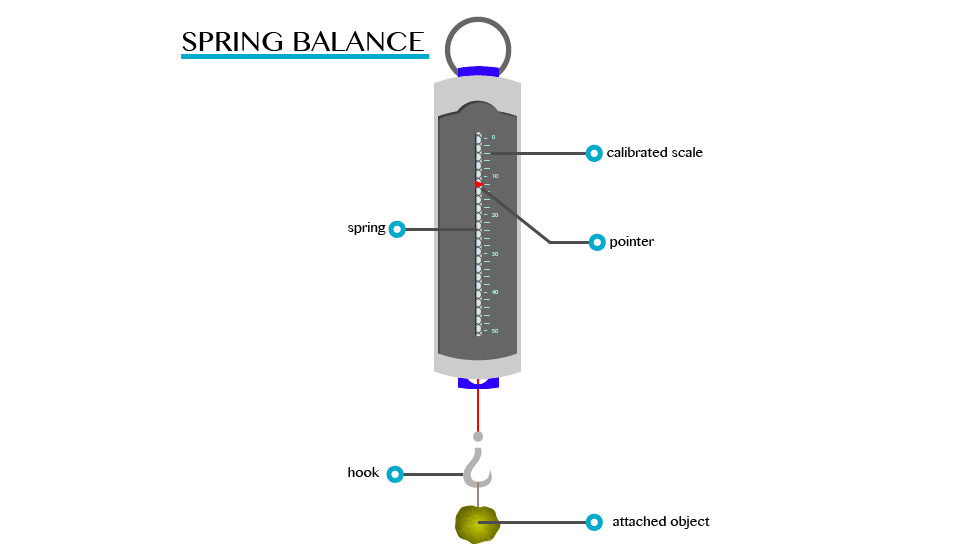# PHYSICS FORM ONE TOPIC 4:FORCE

Posted on

PHYSICS FORM ONE TOPIC 4:FORCE

## SHARE THIS POST ON WHATSAPP

FORCE

A force is an interaction that causes a change. In mechanics, a force is an interaction that causes a change in velocity (an interaction that causes acceleration).
Explain the concept of force
For better understanding of this concept, let’s ask ourselves the following question:
1. What causes/makes a body at rest to move?
2. What causes the same body in motion to stop?
The answers to these questions, is of course that a force is required to do so. Here, an applied force to a body can cause the body at rest to move or if already moving a body application of force can do the following when applied to a body.
1. Force can cause a change in the way the object moves
2. Change its size or shape
3. Change the direction in which an object is moving
A force is defined as a push or pulls experienced by an object. The force is usually described in terms of its size (magnitude) and direction.
The S.I Unit of Force
State the S.I unit of force
The SI unit of force is a Newton (N).
A Newton (N) can be defined as the amount of force required to give a mass of one kilogram (1kg) an acceleration of 1m/s2
Measuring Instrument (Device)
The instrument that is used to measure the amount of force that is exerted on an object is called spring balance.
A spring balance can be used to measure small forces. It consists of a coiled spring fixed to the other end with a hook at the other end. The body upon which the force acts is attached to the hook. The distance through which the spring is stretched is directly proportional to the force applied by the balance.
PHYSICS FORM ONE TOPIC 4:FORCE
A pointer is attached to the spring and the force is indicated on a calibrated scale.A spring balance